Home MATHEMATICS TOPIC 11: PERIMETERS AND AREAS ~ MATHEMATICS FORM 1

TOPIC 11: PERIMETERS AND AREAS ~ MATHEMATICS FORM 1

610
1
SHAREPERIMETERS AND AREAS

The Perimeters of Triangles and Quadrilaterals
Find the perimeters of triangles and quadrilaterals
Perimeter – is defined as the total length of a closed shape.
It is obtained by adding the lengths of the sides inclosing the shape.
Perimeter can be measured in 𝑚𝑚 , 𝑐𝑚 ,𝑑𝑚 ,𝑚,𝑘𝑚 e. t. c
PERIMETERS AND AREAS

Examples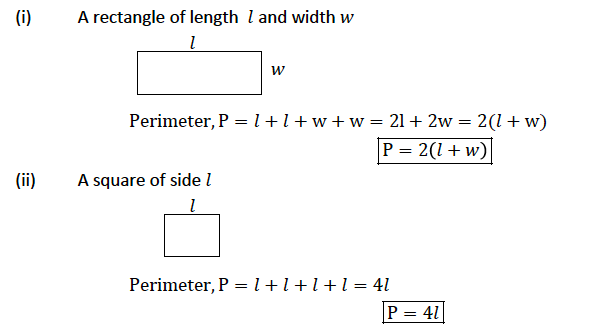PERIMETERS AND AREAS
Example 1
Find the perimeters of the following shapes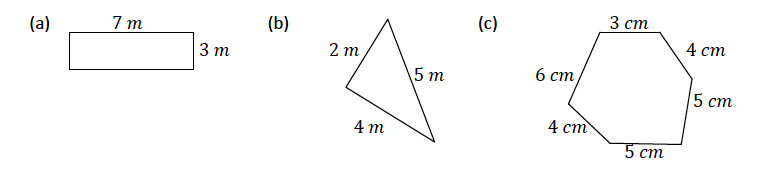Solution
1. Perimeter = 7𝑚 + 7𝑚 + 3𝑚 + 3𝑚 = 20 𝑚
2. Perimeter = 2𝑚 + 4𝑚 + 5𝑚 = 11 𝑚
3. Perimeter = 3𝑐𝑚 + 6𝑐𝑚 + 4𝑐𝑚 + 5𝑐𝑚 + 5 𝑐𝑚 + 4𝑐𝑚 = 27 𝑐𝑚
Areas of Circle
Calculate areas of circle
Consider a circle of radius r;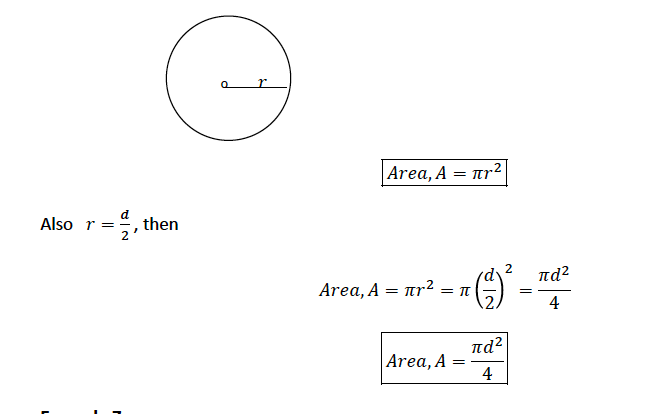PERIMETERS AND AREAS

Example 5

Find the areas of the following figures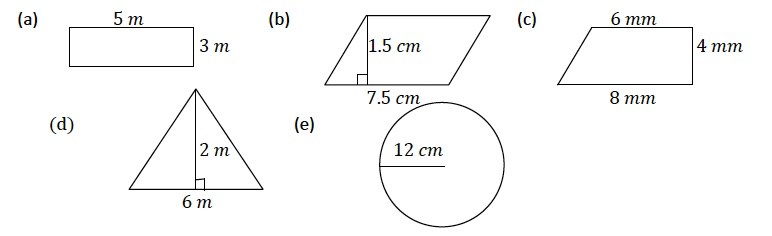Solution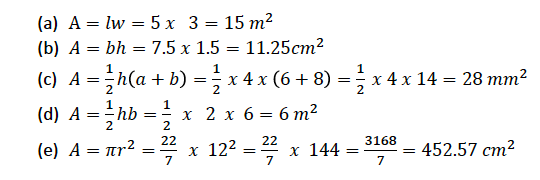PERIMETERS AND AREAS

Example 6

A circle has a circumference of 30 𝑚. What is its area?
Solution
Given circumference, 𝐶 = 30 𝑚
C = 2𝜋𝑟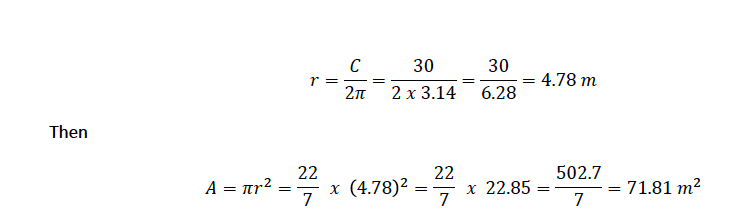SHARE

1 COMMENT

1. Nice I Like That a lot gives you five full star, #teacher hansy kipanya#